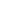Share

# Formula

Example: "A spreadsheet formula can be used to determine the total sum of multiple cells."

A formula is a calculation performed on one or more variables. It may be a simple operation, such as the addition of two numbers, or a complex calculation involving several operations. Formulas are often used within functions to process data within the function. They receive input from the function and return the resulting output. Formulas may also be placed in cells within a spreadsheet to calculate values based on the data stored in other cells.

Published: January 28, 2010

Definition from the PC Glossary
https://pc.net/glossary/formula